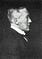Russ Hobbie and I analyze Donnan equilibrium in Chapter 9 of Intermediate Physics for Medicine and Biology.

Section 9.1 discusses Donnan equilibrium, in which the presence of an impermeant ion on one side of a membrane, along with other ions that can pass through, causes a potential difference to build up across the membrane. This potential difference exists even though the bulk solution on each side of the membrane is electrically neutral.

Today I present two new homework problems based on one of Donnan’s original papers.

Donnan, F. G. (1924) “The Theory of Membrane Equilibria.” Chemical Reviews, Volume 1, Pages 73–90.

Here’s the first problem.

Section 9.1

Problem 2 ½. Suppose you have two equal volumes of solution separated by asemipermeable membrane that can pass small ions like sodium and potassium but not large anions like A−. Initially, on the left is 1 mole of Na+and 1 mole of A−, and on the right is 10 moles of K+and 10 moles of A−. What is the equilibrium amount of Na+, K+, and A−on each side of the membrane?

Stop and solve the problem using the methods described in IPMB. Then come back and compare your solution to mine (and Donnan’s).

In equilibrium, x moles of sodium will cross the membrane from left to right. To preserve electroneutrality, x moles of potassium will cross from right to left. So on the left you have 1 — x moles of Na+, x moles of K+, and 1 mole of A −. On the right you have x moles of Na+, 10 — x moles of K+, and 10 moles of A −.

Both sodium and potassium are distributed by the same Boltzmann factor, implying that

[Na+]left/[Na+]right = [K+]left/[K+]right = exp(−eV/kT) (Eq. 9.4)

where e is the elementary charge, V is the voltage across the membrane, k is Boltzmann’s constant, and T is the absolute temperature. Therefore

(1 — x)/x = x/(10 — x)

or x = 10/11 = 0.91. The equilibrium amounts (in moles) are

left right
Na+ 0.09 0.91
K+ 0.91 9.09
A− 1.00 10.00

The voltage across the membrane is

V = kT/e ln([Na+]right/[Na+]left) = (26.7 mV) ln(10.1) = 62 mV .

Donnan writes

In other words, 9.1 per cent of the potassium ions originally present [on the right] diffuse to [the left], while 90.9 per cent of the sodium ions originally present [on the left] diffuse to [the right]. Thus the fall of a relatively small percentage of the potassium ions down a concentration gradient is sufficient in this case to pull a very high percentage of the sodium ions up a concentration gradient. The equilibrium state represents the simplest possible case of two electrically interlocked and balanced diffusion-gradients.

Like this problem? Here’s another. Repeat the last problem, but instead of initially having 10 moles of K+on the right, assume you have 10 moles of Ca++. Calcium is divalent; how will that change the problem?

Problem 3 ½. Suppose you have two solutions of equal volume separated by a semi-impermeable membrane that can pass small ions like sodium and calcium but not large anions like A−and B−−. Initially, on the left is 1 mole of Na+and 1 mole of A−, and on the right is 10 moles of Ca++and 10 moles of B−−. What is the equilibrium amount of Na+, Ca++, A−and B−−on each side of the membrane?

Again, stop, solve the problem, and then come back to compare solutions.

Suppose 2 x moles of Na+cross the membrane from left to right. To preserve electroneutrality, x moles of Ca++move from right to left. Both cations are distributed by a Boltzmann factor (Eq. 9.4)

[Na+]left/[Na+]right = exp(−eV/kT)

[Ca++]left/[Ca++]right = exp(−2eV/kT) .

However,

exp(−2eV/kT) = [ exp(−eV/kT) ]2

so

{ [Na+]left/[Na+]right }2 = [Ca++]left/[Ca++]right

or

[ (1 –2 x)/(2x) ]2 = x/(10 — x) .

This is a cubic equation that I can’t solve analytically. Some trial-and-error numerical work suggests x = 0.414. The equilibrium amounts are therefore

left right
Na+ 0.172 0.828
Ca++ 0.414 9.586
A−1 0
B−− 0 10

The voltage across the membrane is

V = kT/e ln([Na+]right/[Na+]left) = (26.7 mV) ln(4.814) = 42 mV .

I think this is correct; Donnan didn’t give the answer in this case, so I’m flying solo.Frederick Donnan. From an article in the Journal of Chemical Education, Volume 4(7), page 819.

Who was Donnan? Frederick Donnan (1870–1956) was an Irish physical chemist. He obtained his PhD at the University of Leipzig under Wilhelm Ostwald, and then worked for Henry van’t Hoff. Most of his career was spent at the University College London. He was elected a fellow of the Royal Society and won the Davy Medal in 1928 “for his contributions to physical chemistry and particularly for his theory of membrane equilibrium.”

Originally published at http://hobbieroth.blogspot.com.

Professor of Physics at Oakland University and coauthor of the textbook Intermediate Physics for Medicine and Biology.